# Magnetic Force

The magnetic field B is defined from the Lorentz Force Law, and specifically from the magnetic force on a moving charge: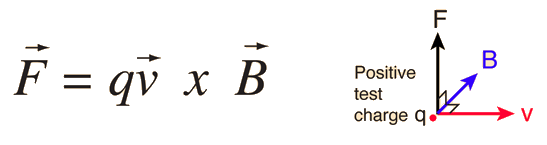The implications of this expression include:

1. The force is perpendicular to both the velocity v of the charge q and the magnetic field B.

2. The magnitude of the force is F = qvB sinθ where θ is the angle < 180 degrees between the velocity and the magnetic field. This implies that the magnetic force on a stationary charge or a charge moving parallel to the magnetic field is zero.

3. The direction of the force is given by the right hand rule. The force relationship above is in the form of a vector product.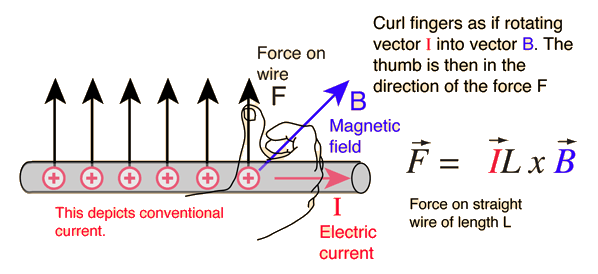When the magnetic force relationship is applied to a current-carrying wire, the right-hand rule may be used to determine the direction of force on the wire.

From the force relationship above it can be deduced that the units of magnetic field are Newton seconds /(Coulomb meter) or Newtons per Ampere meter. This unit is named the Tesla. It is a large unit, and the smaller unit Gauss is used for small fields like the Earth's magnetic field. A Tesla is 10,000 Gauss. The Earth's magnetic field at the surface is on the order of half a Gauss.

 Magnetic interactions with charge Magnetic force applications
 Video: magnetic force on current
Index

Electromagnetic force

Magnetic field concepts

 HyperPhysics***** Electricity and Magnetism R Nave
Go Back

# Lorentz Force Law

Both the electric field and magnetic field can be defined from the Lorentz force law: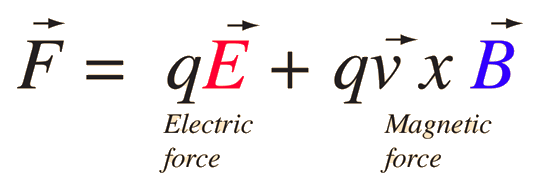The electric force is straightforward, being in the direction of the electric field if the charge q is positive, but the direction of the magnetic part of the force is given by the right hand rule.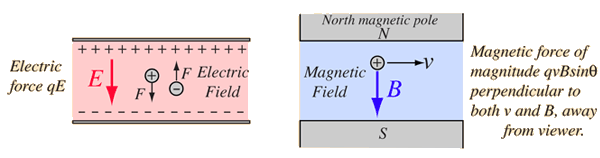Index

Electromagnetic force

Magnetic force

Magnetic field concepts

 HyperPhysics***** Electricity and Magnetism R Nave
Go Back

# Right Hand Rule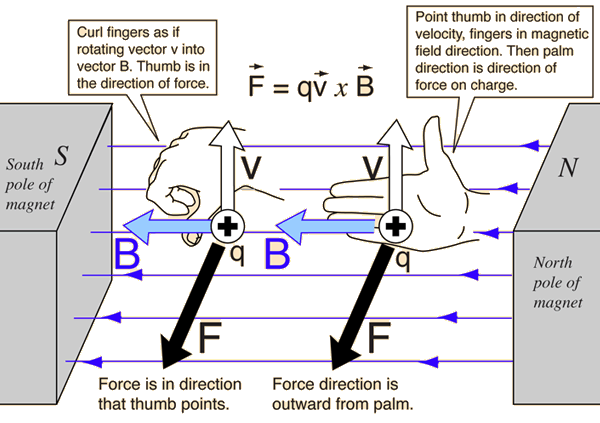The right hand rule is a useful mnemonic for visualizing the direction of a magnetic force as given by the Lorentz force law. The diagrams above are two of the forms used to visualize the force on a moving positive charge. The force is in the opposite direction for a negative charge moving in the direction shown. One fact to keep in mind is that the magnetic force is perpendicular to both the magnetic field and the charge velocity, but that leaves two possibilities. The right hand rule just helps you pin down which of the two directions applies.

For applications to current-carrying wires, the conventional electric current direction can be substituted for the charge velocity v in the above digram.Magnetic force as a vector product
 Magnetic force on a moving charge
Index

Magnetic force

Magnetic field concepts

 HyperPhysics***** Electricity and Magnetism R Nave
Go Back